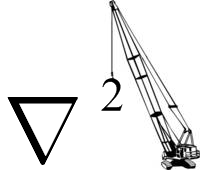## Fusion

Recently, A. Widom of Northeastern University and L. Larsen of Lattice Energy LLC have proposed a theoretical explanation for low energy nuclear reactions (LENR) in the framework of electroweak interactions of ultra-low momentum neutrons. Electroweak interactions are relatively well understood in the Standard Model as the electromagnetic and weak forces are unified in a single field theory. However, their appearance in condensed matter or solid state system is novel, and hence unfamiliar. Most condensed matter interactions are purely electromagnetic: electrons or ions usually have energies in the keV range, and nuclear reactions are absent. Weak interactions, however, such as the capture of an electron by a proton to create a neutron (reverse beta decay), require MeV's of energy to overcome the mass-energy difference, and are usually not observed. However, certain circumstances exist that can accelerate electrons to MeV energies within a condensed matter system, allowing weak interactions (and hence nuclear reactions) to occur.

The standard reaction for electron capture is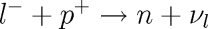whereby any negatively charged lepton can be captured to create a neutron and a neutrino. Note that there is no Coulomb barrier to this interaction; instead the Coulomb attraction actually encourages it, and we can often see this with muons. We do not readily observe the reaction with electrons, however, because of the mass-energy barrier. For the reaction to spontaneously occur, the mass of the lepton (let's deal just with electrons, since they are common and of practical interest) must satisfy a threshold criterion: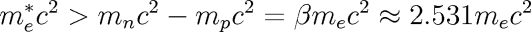We see that the electron's mass must be augmented by a factor of 2.531 to give it enough energy to be captured by a proton. How can we "dress up" the mass of the electron in a condensed matter system? Local electromagnetic field fluctuations!

If we denote the four-momentum of an electron as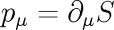in an electromagnetic field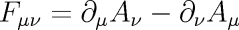we have the Hamilton-Jacobi equation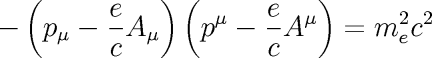Even if the field fluctuations average to zero, the remaining mean square fluctuations can add mass to the electron such that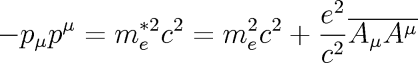Clearly, we must have that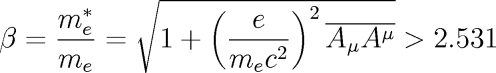for neutron production. As a simplification, we can assume that the rms field fluctuations as a function of position satisfy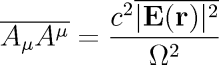so that the local mass enhancement factor is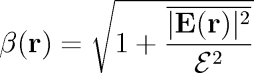where Î© is the frequency scale of the fluctuations, and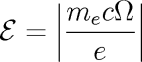is a constant.

To calculate the field strength and proton oscillation frequency, we imagine a small displacement u from equilibrium in a uniform cloud of negative charge, so that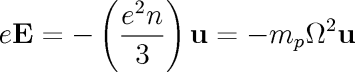where n is the electron density, which we approximate as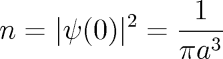for the density at the origin of a hydrogen atom, with a the Bohr radius. With the distinctions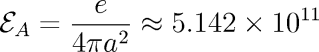for the typical electric field in an atom, and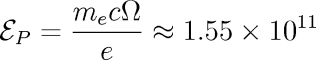for the electric field strength due to the proton oscillations in the condensed matter system (value determined experimentally, both in Volts/meter), we can see that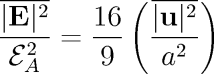giving us a mass enhancement factor of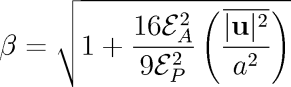Using the values above, we find that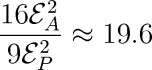so that the electron's mass enhancement is a direct function of the mean square displacement of the protons in their collective mode oscillations.

Under Construction